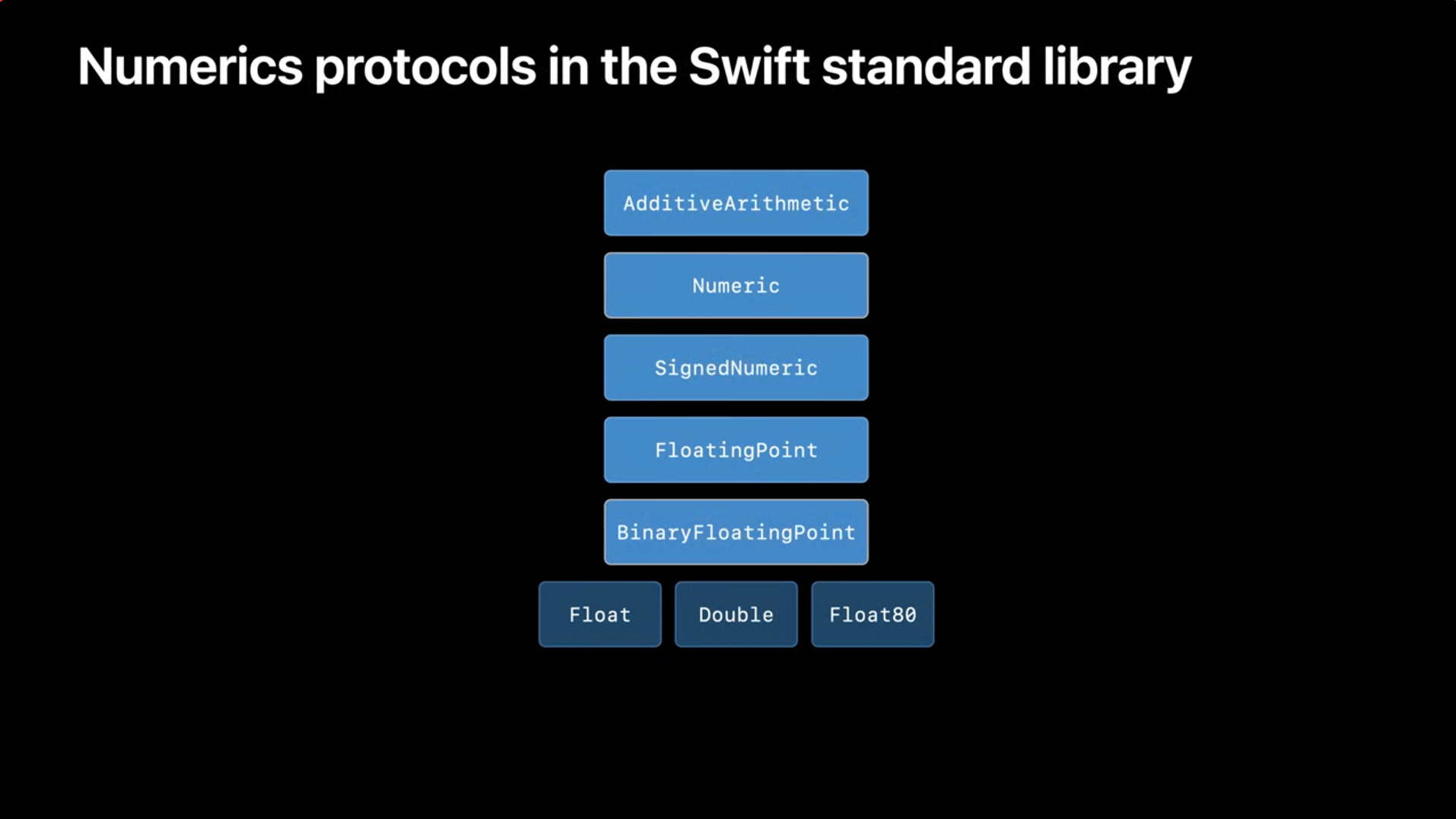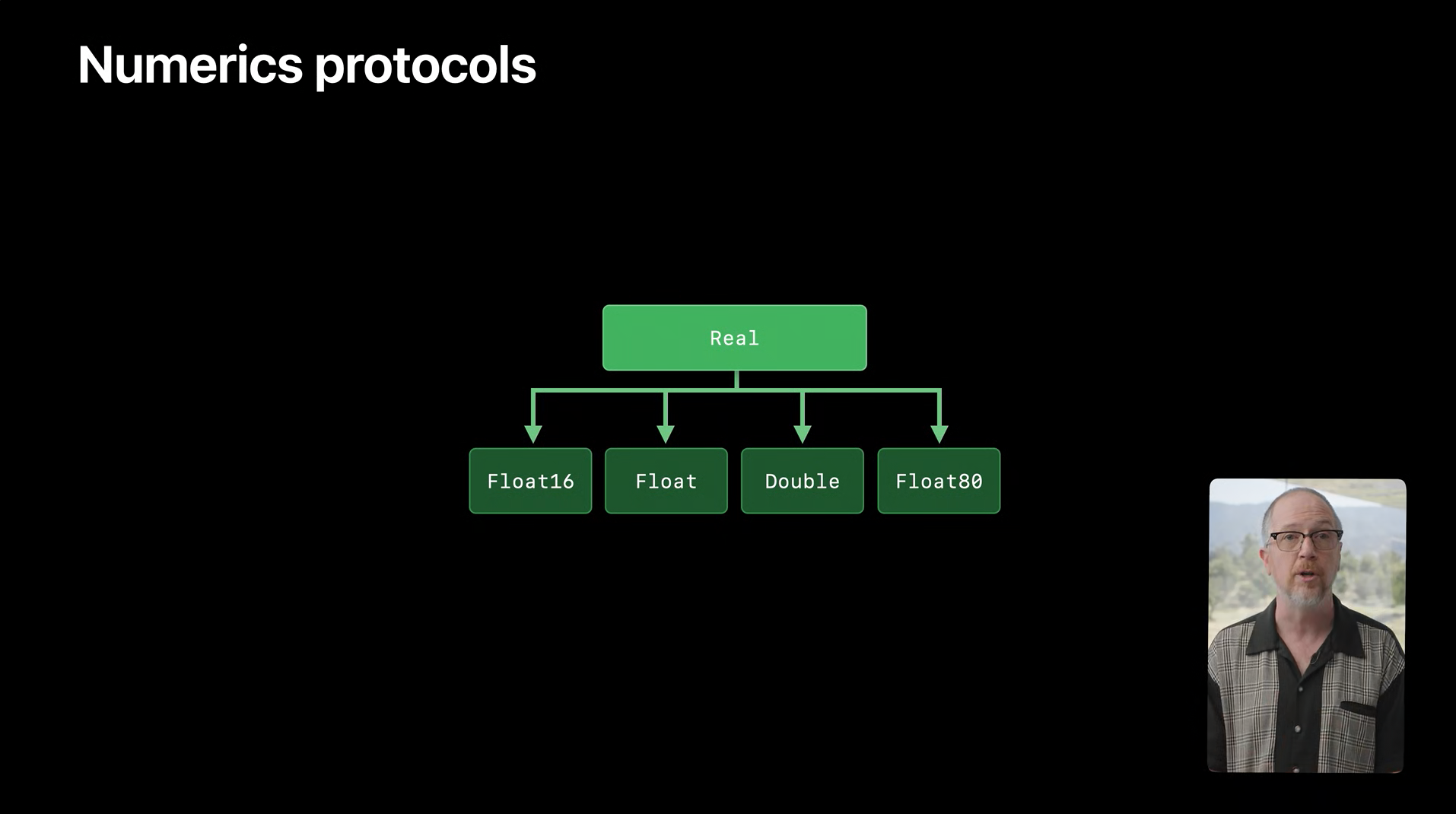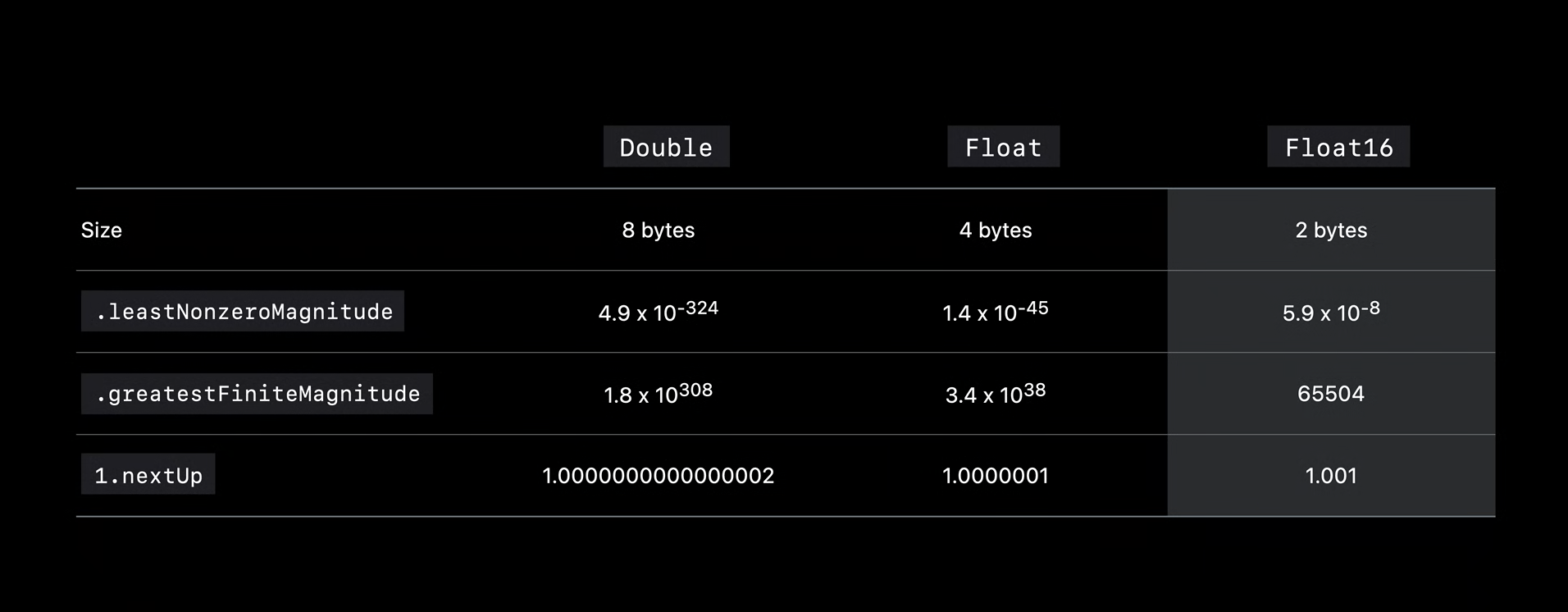WWDC20 Session 10217 - Explore numerical computing in Swift

## Swift Numerics

Numerics 是一个 Apple 开源的 Swift 包，通过范型约束，提供更简单的方式，来使用所有标准库里的浮点型进行数值计算。

import Darwin
/// Logit 模型
///
/// https://en.wikipedia.org/wiki/Logit
///
/// - 参数 p:
///   取值范围 0...1。
///
/// - 返回值:
///   log(p/(1-p))。
func logit(_ p: Double) -> Double {
log(p) - log1p(-p)
}


### Real 协议

Numerics 里面提供了一个全新的 Real 协议，对这类计算的类型提供支持。通过 Real 协议，上面的例子可以改造成：

import Numerics

func logit<NumberType: Real>(_ p: NumberType) -> NumberType {
.log(p) - .log(onePlus: -p)
}


NumberType 范型增加 Real 协议约束，并将 loglog1p 函数替换成 Numerics 里支持范型的 loglog(one plus:) 版本。所有浮点型都会遵循 Real 协议，这个改写后的 logit 函数，不仅能根据平台支持其对应的浮点型参数，在以后标准库增加新的浮点型时，也无需做额外的适配。

public protocol Real: FloatingPoint, RealFunctions, AlgebraicField {
}


Real 协议是一个协议组合，其中 FloatingPoint 协议是标准库中的协议，其余两个协议是 Numerics 里所提供的新协议。这里需要注意的是，对于开发者而言，只应该使用 Real 协议本身• SignedNumeric：拓展了乘法概念。
• FloatingPoint：拓展了计算机中浮点型实现所需要的各种概念，比如比较、幂运算和有效位数等，还有各种常用的变量 infinity（∞）、nanpi 等。

### AlgebraicField 协议

public protocol AlgebraicField: SignedNumeric {
static func /(a: Self, b: Self) -> Self

/// 倒数
var reciprocal: Self? { get }

/// ...
}


SignedNumeric 的基础上拓展了除法概念。这样就支持了全部四则运算，数学领域称为”代数数域“，这也是这个协议名字的由来。

### ElementaryFunctions 协议

public protocol ElementaryFunctions: AdditiveArithmetic {
/// 指数
static func exp(_ x: Self) -> Self

/// exp(x) - 1
static func expMinusOne(_ x: Self) -> Self

/// 三角函数
static func cos(_ x: Self) -> Self
static func sin(_ x: Self) -> Self
static func tan(_ x: Self) -> Self

/// 对数
static func log(_ x: Self) -> Self

/// log(1 + x)
static func log(onePlus x: Self) -> Self

/// exp(y * log(x))
static func pow(_ x: Self, _ y: Self) -> Self

/// 幂
static func pow(_ x: Self, _ n: Int) -> Self

/// 次方根
static func root(_ x: Self, _ n: Int) -> Self

/// ...
}



AdditiveArithmetic 的基础上拓展了大量通用的浮点型函数，包括核心的三角函数、指数、对数、幂和次方根等。

### RealFunctions 协议

public protocol RealFunctions: ElementaryFunctions {
/// 误差函数
static func erf(_ x: Self) -> Self

/// sqrt(x*x + y*y)
static func hypot(_ x: Self, _ y: Self) -> Self

/// Γ(x)
static func gamma(_ x: Self) -> Self

/// log(|Γ(x)|)
static func logGamma(_ x: Self) -> Self

/// ...
}


ElementaryFuctions 的基础上拓展了更多类似但少用的函数，比如伽马函数、误差函数和更多底数的指数和对数等。

• 范型支持

• 解决重复的代码

• 更低的维护成本

• 更好的兼容性（支持新的浮点型）

### Complex 类型

Complex 类型是 Numerics 中的一部分，为 Swift 提供了复数支持，且是使用 Real 协议作为范型约束的。

import Numerics

let z = Complex(1.0, 2.0) // z = 1 + 2 i，这里默认是 Double


Complex 类型不仅本身很好用，同时也是一个使用 Real 协议进行范型数值编程的好范例。

/// 定义 NumberType 遵循 Real 协议
public struct Complex<NumberType> where NumberType: Real {
/// 实数部分
public var real: NumberType

/// 虚数部分
public var imaginary: NumberType

/// ...
}


extension Complex: SignedNumeric {
public static func +(z: Complex, w: Complex) -> Complex {
return Complex(z.real + w.real, z.imaginary + w.imaginary)
}

public static func -(z: Complex, w: Complex) -> Complex {
return Complex(z.real - w.real, z.imaginary - w.imaginary)
}

public static func *(z: Complex, w: Complex) -> Complex {
return Complex(z.real * w.real - z.imaginary * w.imaginary,
z.real * w.imaginary + z.imaginary * w.real)
}
}


extension Complex {
/// 长度
public var length: NumberType {
return .hypot(real, imaginary)
}

/// 相位角
public var phase: NumberType {
return .atan2(y: imaginary, x: real)
}

public init(length: NumberType, phase: NumberType) {
self = Complex(.cos(phase), .sin(phase)).multiplied(by: length)
}
}


Complex 类型是一个扁平的结构体，包含着两个浮点型的值。这样，和 C（_Complex double） 与 C++ （std::complex<double>）里的复数类型有着精确匹配的内存布局。这使得 Swift 的复数和 C/C++ 有互操作的可能。在 Swift 中创建的复数缓冲区，可以通过指针传递给 C/C++ 的库使用。

import Numerics
import Accelerate

/// 100 个随机的复数
let z = (0 ..< 100).map {
Complex(length: 1.0, phase: Double.random(in: -.pi ... .pi))
}

/// 计算 L2 范数（欧几里得范数）
let norm = cblas_dznrm2(z.count, &z, 1)


• 改进的整型幂运算
• 近似相等的新处理工具

## Float16 类型

Float16 是 Swift 标准库中新增的数据类型，顾名思义占用 16 位（2 字节）。

• IEEE 754 标准格式
• 基于 x86 的平台正在支持中（和 Intel 在一起修复中）

Float16 是一个完整支持的标准浮点型。

• 遵循 BinaryFlatingPointSIMDScalar
• 遵循 Numerics 的 Real
• 支持所有的标准浮点型函数• 更好的性能
• 与 C/Objective-C 里 __fp16 类型的互操性

• 低精度和小范围• Apple GPU 已经支持（且为偏向选择）
• Apple CPU 从 A11 Bionic 之后开始已经选择
• Scalar（标量）性能与 Float/Double 相同
• SIMD 性能 2x 于 Float
• 更老的 CPU 通过（用 Float 操作）模拟支持

## 最后

Float16 加入标准库，让 Swift 本身选择余地更多，可以踏足的领域更加丰富。

• 开源开放
• 多平台支持
• 性能出众
• 和 C 良好的互操性